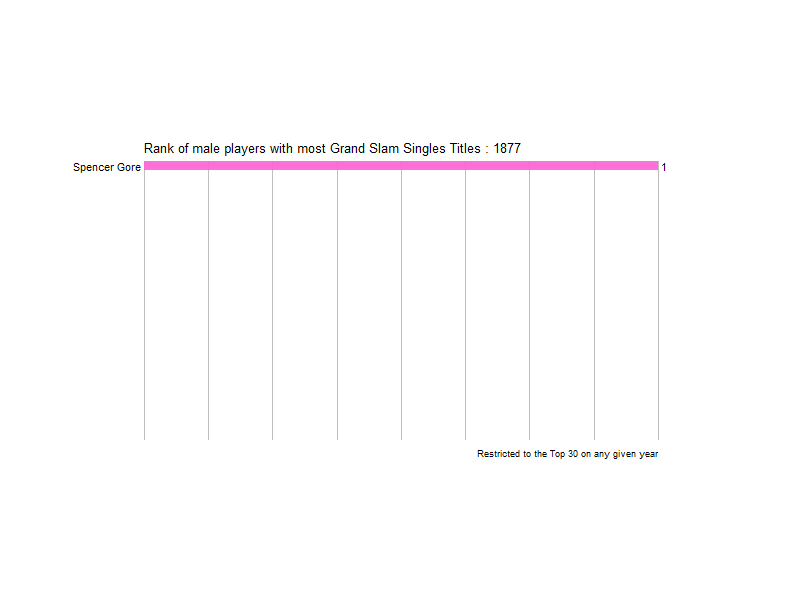# Grand Slam title history as an animated bar chart race

May 16, 2020 `R` `tennis` `gganimate` `ggplot`I’ve spoiled it by putting the gif at the start of the post but if you are interested in how it was made then read on!

I’ve seen this kind of charts around the web so I wanted to make a tennis-related one and what better than using Grand Slam wins since the very beginning; 1877.

The main package that is needed for the animation is gganimate. As the name suggests it integrates with ggplot to make an animation given many different charts and a transition variable.

``````require(XML)
require(data.table)
library(httr)
require(dplyr)
require(stringr)
require(ggplot2)
require(gganimate)
``````

Then we need to get the data for the chart. Wikipedia helpfully has an article with all Grand Slam winner in history so we can pull the table within the article using `GET` and `readHTMLTable`

Once we know where the table is located in the HTML we can pull it into a data table.

There are some kinks in the table we have to get rid off. For example, in 1977 there were two Austrlian Opens so the entry for 1977 is split into two rows but just one year.

We then get rid of anything that is not a player name including special characters. Tjem the table is melted so we get one entry per year and Grand Slam.

We also get rid of other stuff such as all the French Opens before 1925 because the tournament was not actually “open” and also instances when the tournaments were not held such as world wars.

``````gs<-data.table(tabs[])

names(gs) <- as.character(unlist(gs[1,]))
gs<-gs[-1]

gs<-bind_rows(gs,data.table(Year="1977","Australian Open"="Vitas Gerulaitis"))

gs<-gs[grep("[0-9]",Year)][order(Year)]

gs <- melt(gs, id.vars = "Year")

gs\$winner <- gsub("\\(([^)]+)\\)","",gs\$value)

gs\$winner<-gsub("[*]","",gs\$winner)
gs\$winner<-gsub("[†]","",gs\$winner)

gs\$winner<-gsub("Amateur Era ends","",gs\$winner)
gs\$winner<-gsub("Open Era begins","",gs\$winner)

gs[,winner:=str_trim(winner)]

gs[,.N,winner][order(-N)]
``````
``````##                      winner  N
##   1: tournament not created 43
##   2:                   <NA> 24
##   3:          Roger Federer 20
##   5:         Novak Djokovic 17
##  ---
## 167:           Rafael Osuna  1
## 168:         Manuel Orantes  1
## 169:           Andy Roddick  1
## 170:  Juan Martín del Potro  1
## 171:            Marin Cilic  1
``````
``````gs<-gs[!(variable=="French Open" & Year<1925)]

gs[,win:=1]

gs<-gs[!grep("tournament|started|WorldW|occupation|Tournament|oronavir",winner)]

gs<-gs[winner!=""]
``````

We now need to keep a running tally of anyone who has won at least one Grand Slam for every year so that they show up in our chart with the correct number of GS’s. This is what the `fun` function is doing below.

Additionally we also need to rank the players from most GS’s to least GS’s to create a rank variable.

``````#Get a list of all the years
yearList<-gs[order(Year)][,unique(Year)]
#Function fun calculates cumulative GS wins for all the players up to the current year
fun<-function(year){ gs[Year<=year,.(win=sum(win),latestWin=max(Year)),.(winner)][,year:=year] }
#Create a table that has all combinations of year/player
gsfull<-lapply(yearList, fun) %>% rbindlist()

gsfull<-gsfull[order(year,-win,-latestWin)]
gsfull[,rank:=seq(1,.N),year]

gsfull[,win_label := paste0(" ", win)]
``````

We can now start plotting our data. First create a tile plot with ggplot. Tiles work better than plot for this case because they slide into position in a nicer way when the plot transitions between years.

A lot of the code I’m using I found over here in stack overflow.

``````y<-1877

sp<-ggplot(gsfull[year>=y & rank<=30],aes(x=rank,y=win,fill=winner)) +
geom_tile(aes(y=win/2,height=win, width=0.95),alpha=0.9) + theme_bw() +
geom_text(aes(y=0,label = paste0(winner," ")), hjust = 1) +
geom_text(aes(y=win,label = win_label, hjust=0)) +
coord_flip(clip = "off", expand = F) +
scale_x_reverse() +
guides(color = FALSE, fill = FALSE) +
theme(axis.line=element_blank(),
axis.text.x=element_blank(),
axis.text.y=element_blank(),
axis.ticks=element_blank(),
axis.title.x=element_blank(),
axis.title.y=element_blank(),
legend.position="bottom",
panel.background=element_blank(),
panel.border=element_blank(),
panel.grid.major=element_blank(),
panel.grid.minor=element_blank(),
panel.grid.major.x = element_line( size=.1, color="grey" ),
panel.grid.minor.x = element_line( size=.1, color="grey" ),
plot.margin = margin(5,5,5,5, "cm")
)
``````

With the base plot made it’s time to actually animate it. This is where `gganimate` comes into play. The main funtion needed is `transition_states` which takes a transition parameter, `year` in our case, and animates the plot based on it.

There’s a few extra bits in there; `enter_drift` and `exit_shrink` govern how the bars enter and leave the plot and `ease_aes` controls how the bars switch around. There are many other options that `gganimate` provides so this is just scratching the surface.

``````p <- sp + transition_states(year, transition_length = 4, state_length = 2) +
view_follow(fixed_x = TRUE)  +
labs(title = 'Grand Slam Titles : {closest_state}')  +
enter_drift(y_mod=10) + exit_shrink()  +
ease_aes('linear')
``````

Finally once the transitions are defined `animate` takes the object and turns it into a gif or a video if you want depending on the renderer that you choose. The code below is what renders the plot at the start of this post.

Duration and size parameters are passed by the user. Here I would like to note that if you call the plot `p` it does get rendered but it looks different than the output you get with animate so I’d recommend always running `animate` to see what the actual final output will be.

``````animate(p, 1200, fps = 10,  width = 800, height = 600,
renderer = gifski_renderer("gs_chart.gif"))
``````

And that’s it! As with most projects the trickiest part was getting the data in the format I needed it and then spent some time with aesthetic choices. The point being that once you have your data ready `ggplot` and `gganimate` provide an intuitive framework to create cool looking charts.